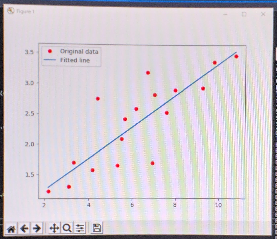## Google’s Solution to Demystifying the AI BlackBox Problem

Nov.25.2019

Author　：Justin Brunnette

Category： IT News

For those of us that are undertaking the creation of AI and machine learning system, we run into the problem of the infamous AI “blackbox”. This is essentially the hidden algorithm inside of the machine learning system that creates the prediction results but the engineers themselves do not know how exactly they work themselves.

This was a problem that services like Youtube was facing when they could not necessarily explain how recommendation algorithm made the various decisions that it did. But Google has stepped up to the plate to take on this problem. They have developed a service called AI Explainable which will help ML engineers better understand what  is going on under the hood.

ML development, such as in the case with Google’s deep learning system TensorFlow, initially takes in large number of data sets to train its algorithm. In a simple two variable system, engineers would then need to extract numeric data from each of these data points, such as rgb values for individual pixels on an image. They would then plot all the points on a graph to calculate a regression line to first get a best fit line for the trend in the data.

They will then compare the actual data point with the corresponding point in the regression line, and the difference between the points is what is called an error. When the actual data point is above the regression line it is positive error and when it is below the line, it is a negative error. Gathering all the points will get you the mean square error of your graph. The closer the mean square error is to zero, the better the regression line fits the data.Above is a sample graph from the AI program have constructed.
The blue line in the simple linear regression and the red dots are the data points. The distance between the regression line and plots are the "errors".

In order to optimize the model, all the errors are gathered and plotted on an additional graph, which will give you a polynomial equation. ML system will need to figure out how to find the global minimum of the graph. In order to do this, it will need to use an optimization algorithm such as gradient descent optimizer or Adam optimizer. When the algorithm finds the global minimum, this becomes the slope of the regression line and a new regression line is created and the system can check the accuracy of the with calculating the mean square error and restart the aforementioned process.

When new data points are added to the system, the system will also make a new regression line and start the aforementioned process. ML are not fed all the data at once but rather in pools of data. Each pool of data is seen as a layer and the ml algorithm will make connections between various nodes or “neurons” across the algorithm. There is also commonly a dropout of neurons during training that will force the network to develop patterns across all neurons. This will make the algorithm become exceedingly more complicated with each new layer introduced to the point that the engineer can no longer understand what the underlying code is doing to get the results  that it is doing.

So essentially it is easier to get the ML system up and running and to get the end result than to understand how the resulting algorithm works. This is the “blackbox” that develops when creating an ML system and can cause problems for businesses when they need to understand what kinds of biases are appearing in the system and if they want to try to adjust those biases.

How Google tackles this problem is to integrate “feature attributions” to the AI prediction. Features are the variables that an algorithm takes into consideration when developing its predictions. In the case with image recognition, these would be the outline pattern of the image, the contours of the image, the coloration etc. When the prediction is made, these features will be attributed a score on how they were used in the prediction. This will help explain which features were used in the prediction and how strongly they were considered when making the prediction.

AI Explainable calculates these feature attributions by using things called counterfactuals, which are predictions that do not lead to the result, which are compared with the actual result. It essentially asks why the model made this prediction rather than the other. By comparing numerous coutnerfactuals, it will start to get a sense of what the model is not paying attention to when making the predictions.

This is a practical step forward for businesses and research centers to analyze, debug and improve upon their models. But to be clear, this would not provide a complete understanding of the underlying code of the ML model. Truely understanding the black box is a frontier in the field that competitors such as Microsoft and Amazon are competing against.

A more detailed explanation is provided in Google’s white paper: